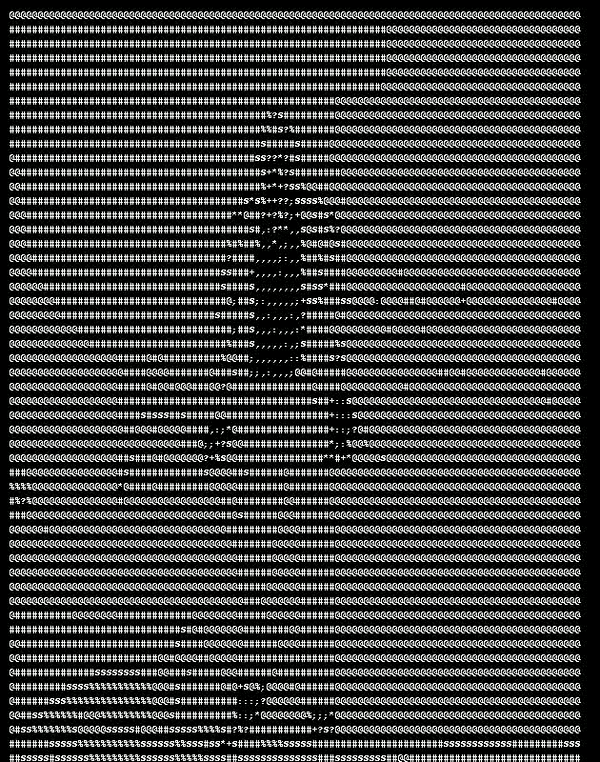top of page
Search
• Andrew Jones

# Asciify yourself using Python (Part 2: Video)

In the previous post, we looked at using Aditya Ramesh's Python script to convert a picture into ASCII symbols.

It's worth having a look at Aditya's Github page, and getting the required code for converting images, then we'll run through how we applied that to video.

Now, we'll look to go one step further and apply this over a video!First, let's import the PIL packages we need, as well as numpy and OpenCV

######################################################################

# import packages

######################################################################

from PIL import Image, ImageDraw, ImageFont

import cv2

import numpy as np

Next, we specify the input video that we want to convert, and the name of the file we'll save the converted video to. We also want to specify the width of the image (we re-size it and may want to play around with this)

######################################################################

# parameters

######################################################################

VIDEO_IN = moonwalk_edited.mp4'

VIDEO_OUT = moonwalk_out.mp4'

NEW_WIDTH = 100

This is straight from Aditya's original code, it's the list of ASCII characters, put into a list

######################################################################

# ascii character list

######################################################################

ASCII_CHARS = ['.',',',':',';','+','*','?','%','S','#','@']

ASCII_CHARS = ASCII_CHARS[::-1]

Again, these are the functions out of the original code

######################################################################

# functions

######################################################################

######################################################################

# resize

######################################################################

def resize(image, new_width=NEW_WIDTH):

(old_width, old_height) = image.size

aspect_ratio = old_height/old_width

new_height = int(aspect_ratio * new_width)

new_dim = (new_width, new_height)

resized_image = image.resize(new_dim)

#new_image = new_image.convert('L')

return resized_image

######################################################################

# grey-scale

######################################################################

def grayscalify(image):

grey_image = image.convert('L')

return grey_image

######################################################################

# replace every pixel with a character whose intensity is similar

######################################################################

def modify(image, buckets=25):

initial_pixels = list(image.getdata())

new_pixels = [ASCII_CHARS[pixel_value//buckets] for pixel_value in initial_pixels]

return ''.join(new_pixels)

######################################################################

# compile all steps

######################################################################

def process_image(image, new_width=NEW_WIDTH):

image = resize(image)

image = grayscalify(image)

pixels = modify(image)

len_pixels = len(pixels)

# construct the image from the character list

new_image = [pixels[index:index+new_width] for index in range(0, len_pixels, new_width)]

return '\n'.join(new_image)

Now, this is the new code...

If you're familiar with OpenCV then this should make sense, otherwise it could be worth getting to grips with that first - although I'm sure you can follow along.

Firstly, we use OpenCV's VideoCapture function and pass it the video file we specified above.

We then set up the video codec we want to use to write our processed video to disk. This needs the location of where we want to save the file, as well as the Frames per Second, and the width and height we want for the video.

Within a while loop, we then process each frame of this video. We run it through our Asciify functions above.

Then, we create a blank canvas, as we need to turn the symbols into an image. We then write the symbols onto the canvas using the PIL package's Draw and Text functionalities.

We then need to convert this to a numpy array to be able to write the frames into a video file.

cv2.imshow means we can watch the resulting video in real-time during the while loop.

The last four lines of the code are very specific and unique to OpenCV, I won't go into detail here

######################################################################

# convert the video, and save

######################################################################

cap = cv2.VideoCapture(VIDEO_IN)

# define the codec and create VideoWriter object

fourcc = cv2.VideoWriter_fourcc(*'XVID')

writer = cv2.VideoWriter(VIDEO_OUT,fourcc, 30, (630,800))

while True:

# convert from numpy array to PIL image

frame_img = Image.fromarray(frame)

image_symbols = process_image(frame_img)

canvas = Image.new('RGB', (630, 800), color = (0, 0, 0))

d = ImageDraw.Draw(canvas)

d.text((10,10), image_symbols, fill=(255,255,255))

canvas1 = np.array(canvas)

# write the frame

writer.write(canvas1)

cv2.imshow('frame',canvas1)

if cv2.waitKey(1) & 0xFF == ord('q'):

break

cap.release()

cv2.destroyAllWindows()

I hope you were able to have a play around with this. I can't think of any genuine reasons you want to do this, but it's quite an interesting concept at the very least!